# Archimedean class

(diff) ← Older revision | Latest revision (diff) | Newer revision → (diff)

A class resulting from the subdivision induced by the Archimedean equivalence relation on a totally ordered semi-group. This equivalence is defined as follows. Two elementsand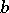of a semi-groupare called Archimedean equivalent if one of the following four relations is satisfied: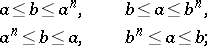which amounts to saying that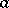andgenerate the same convex sub-semi-group in. Thus, the subdivision into Archimedean classes is a subdivision into pairwise non-intersecting convex sub-semi-groups. Moreover, each subdivision into pairwise non-intersecting convex sub-semi-groups, can be extended to a subdivision into Archimedean classes.

The Archimedean equivalence on a totally ordered group is induced by the Archimedean equivalence of its positive cone: It is considered that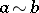if there exist positive integersandsuch that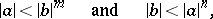whereThe positive cone of an Archimedean group consists of a single Archimedean class.

How to Cite This Entry:
Archimedean class. Encyclopedia of Mathematics. URL: http://encyclopediaofmath.org/index.php?title=Archimedean_class&oldid=11365
This article was adapted from an original article by O.A. Ivanova (originator), which appeared in Encyclopedia of Mathematics - ISBN 1402006098. See original article